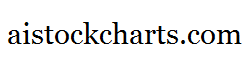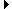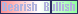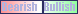Members AreaSign Up | Log In
 Free Level 2 QuotesStock AnalysisCorrelation Trading PlatformCorrelationsMembership Benefits

P5RANGE
 Lookup a different indicator.

 Indicator Usage Description Example p5range p5range[n] OPERATOR (INDICATOR or POSITIVE NUMBER) , where n is a positive integer >= 0. Returns the percentage change from the lowest price among day n, day n-1, day n-2, day n-3 and day n-4 to the highest price among day n, day n-1, day n-2, day n-3 and day n-4 where n=0 is the last trading day, n=1 is the previous day, etc. (p5range < 3)

Introduction:

The 'p5range' indicator is used in indicator formula construction to narrow the stock pre-screener results to include only those stocks that have a percentage change from the lowest to highest price of 5 consecutive days that are restricted to the parameters set for the p5range indicator.

Example:

The chart of Intel (INTC) below highlights days where the percentage change from the lowest to highest stock price of 5 consecutive days was less than 3%.

 Chart of Intel (INTC) highlighting days where the percentage change from the lowest to highest price of 5 consecutive days was less than 3%.

INDICATORS IN OUR DATABASE THAT INCLUDE THE P5RANGE INDICATOR...

Click here to view all indicators that include the indicator 'p5range' (sorted by AISCORE)

 (p5range < 3)AISCORE: 1374Indicator ID: 060131092635(close < sma) and (close > sma) and (vol > 1.1 * vol) and (p5range < 14)AISCORE: 1122Indicator ID: 060519103620(close < sma) and (close > sma) and (vol > 1.2 * vol) and (p5range < 15)AISCORE: 1041Indicator ID: 060519103722(close < sma) and (close > sma) and (vol > 1.15 * vol) and (p5range < 15)AISCORE: 1252Indicator ID: 060519103811(close < sma) and (close > sma) and (vol > 1.15 * vol) and (p5range < 15)AISCORE: 244Indicator ID: 060519103901 More ->